# User:IssaRice/Computability and logic/K is recursively enumerable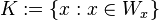$K := \{x : x \in W_x\}$

## Proofs

### Finding a partial recursive function that enumerates K

This proof is from Enderton.

Proof. Let$\phi_0, \phi_1, \phi_2, \ldots$ be a standard enumeration of the partial recursive functions.

We can define the diagonal partial function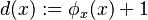$d(x) := \phi_x(x) + 1$. This is a partial recursive function because both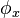$\phi_x$ and$x \mapsto x+1$ are, and we can obtain$d$ via substitution. Thus,$d = \phi_e$ for some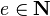$e \in \mathbf N$. (Actually, is it really so simple to show$d$ is partial recursive? We're not just using a fixed$\phi_x$, but rather we are allowing the partial function index to move around. To be more rigorous, I think we need to say that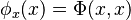$\phi_x(x) = \Phi(x,x)$, where$\Phi$ is the two-place universal function. Since the universal function is partial recursive, we can be sure that we can use the expression "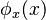$\phi_x(x)$" and move the index around. Even more formally, we can say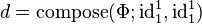$d = \mathrm{compose}(\Phi; \mathrm{id}^1_1, \mathrm{id}^1_1)$.)

But now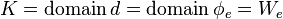$K = \operatorname{domain} d = \operatorname{domain} \phi_e = W_e$. Since a set is recursively enumerable iff it is the domain of some partial recursive function, this shows that$K$ is recursively enumerable.

Discussion. The definition of$d$ doesn't seem to be important. Actually, I think we can just take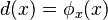$d(x) = \phi_x(x)$. But it seems Enderton did the plus one to make a further point (namely, that it looks like we might be diagonalizing out of this class of functions, but luckily we're not, thanks to our objects only being partial functions).

### Using programs

Peter Smith. An Introduction to Gödel's Theorems. p. 24.